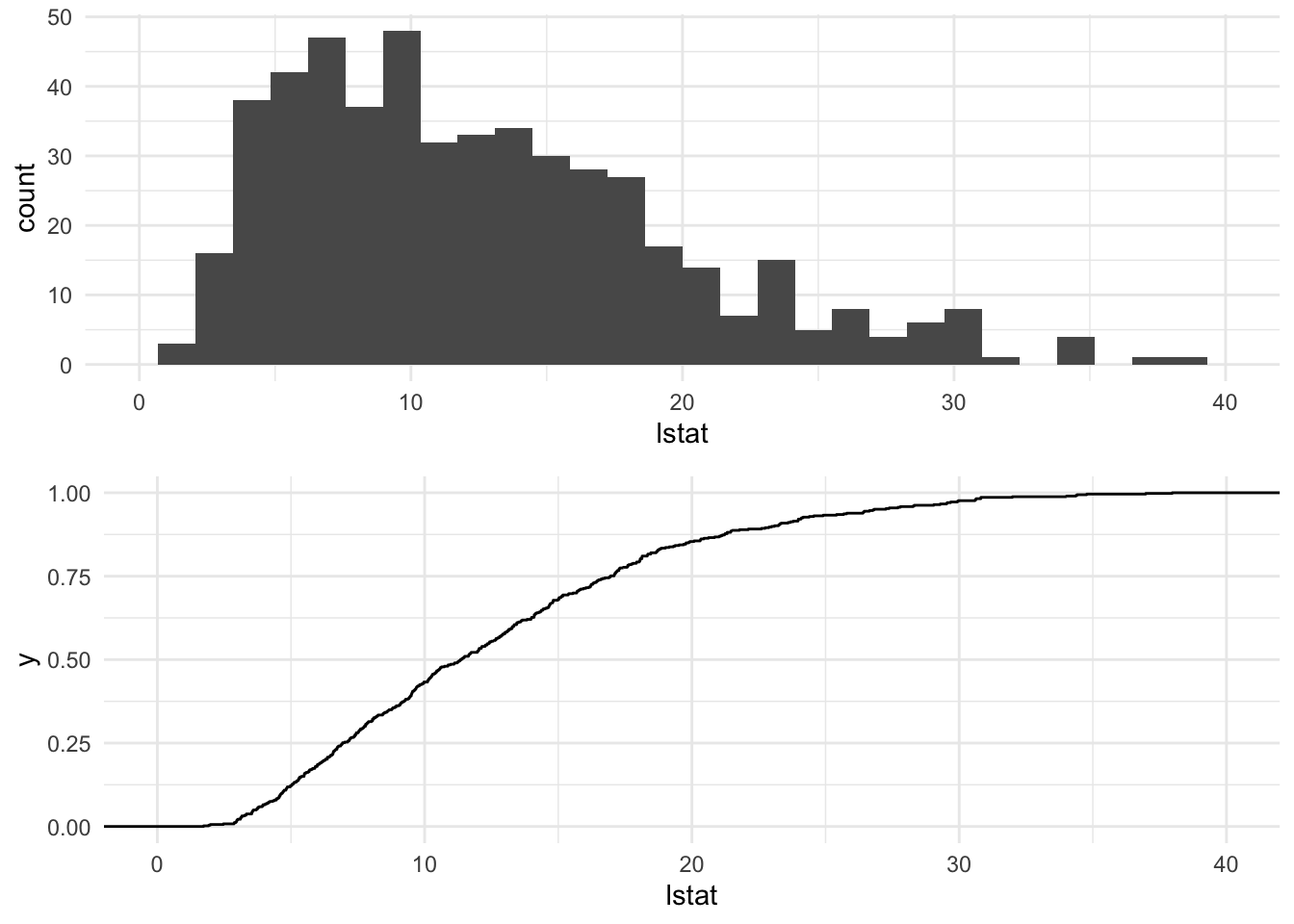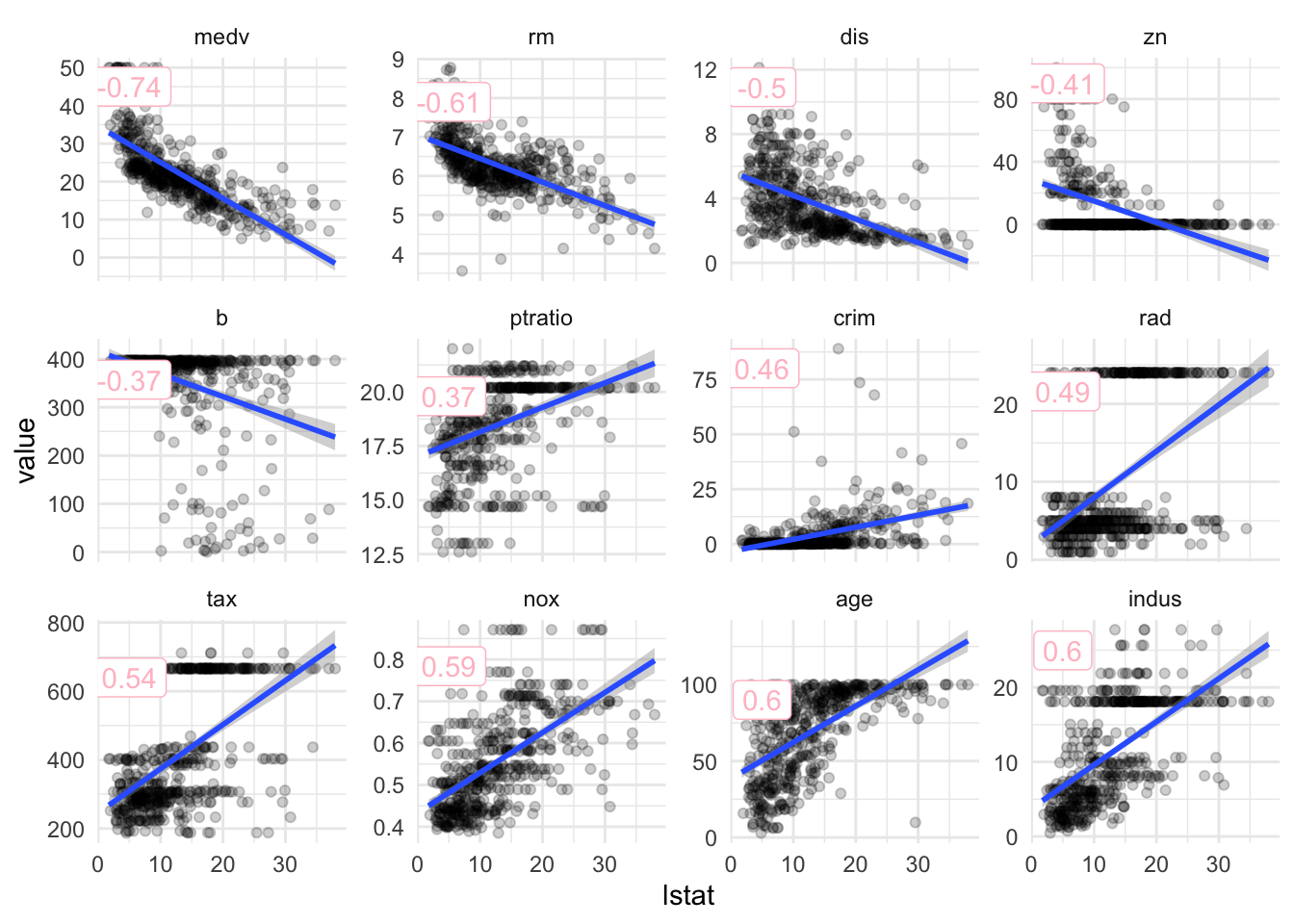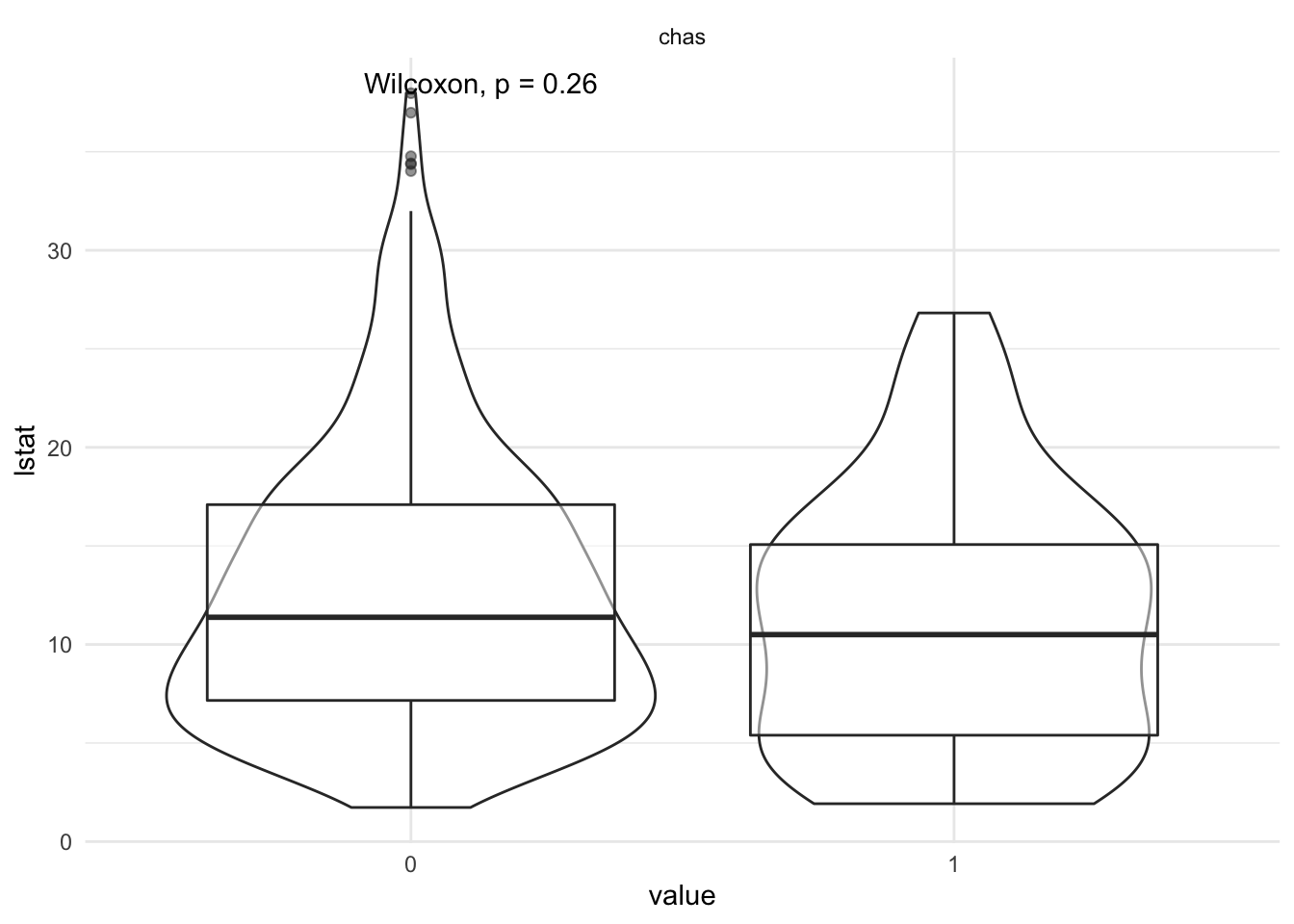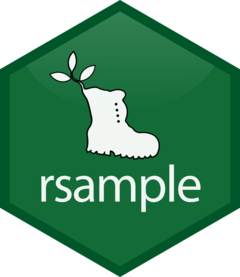# Introduction

RStudio is expanding the `tidyverse` principles to modelling with R and is building up another metapackage called `tidymodels`. There are a number of packages at different stages in their development. I am already familiar with `rsample` and `recipes` and have tried to implement them in a tidy `caret`-based modelling workflow before.

The goal of this post is to check up on all the different packages and try build up a regression modelling workflow using all the appropriate `tidymodels` tools as soon as they become available at CRAN for I think that this indicates that the authors were confident enough that their package has reached an acceptable stage of maturity.

# Packages

## CRAN availability of tidymodels packages:

package CRAN description
broomConvert statistical analysis objects from R into tidy format
rsampleClasses and functions to create and summarize different types of resampling objects
dialsTools for creating tuning parameter values
textrecipesExtra recipes for Text Processing
yardstickTidy methods for measuring model performance
parsnip NR A tidy unified interface to models
probably NR Tools for post-processing class probability estimates
recipesA preprocessing engine to generate design matrices
embedExtra recipes for categorical predictor embeddings
inferAn R package for tidyverse-friendly statistical inference
tidyposteriorBayesian comparisons of models using resampled statistics

## Unified Modelling Syntax

The declared goal of the `tidymodels` metapackage is to provide a unified modelling synthax similar to `scikit-learn` in the `python` domain or an improved version of `caret` but adhering to the `tidyverse` principles. `parsnip` is going to be the core package while `dials` will provide suitable objects and functions for parameter tuning. The amount of supported models is still a bit meager so we will not explore these packages any further for the moment.

## Statistical Tests and Model Selection

The regular statistical test supported by `R` have the same problem as the modelling implementations, they lack a uniform `tidyverse` compatible synthax. Further traditional statistical tests have lately gotten a bit out of fashion. The following criticism keeps popping up:

• Specific statistical requirements for each test. The strategies for selecting the right statistical tests are a bit convoluted and a certain set of statistical requirements need to be full-filled for each of them.

• Interpretation of P Values. There is a pickiness when it comes to interpreting P Values, the perfect definition eludes me and is completly useless to a none-statistician. Allen Downey has a refreshing practical approach to P values in which he uses a bayesian approach to show that indeed from small p values (<= 0.01) one can conlude that the observed effect has a low probability to be the result of chance (post)

• Disregard of Effect Size. If we have a large sample even irrelevant effects will result in low p-values and if we have a small sample only very large effects will result in low p-values. If we detect a relevant effect with a low p-value we cannot be sure that the magnitude of the effect is reproducible. Typically the effect size will decrease the larger the sample. The Null hypothesis does not incorporate a minimum effect size.

As a remedy for the issue of the convoluted statisical requirements for each test a workaround has again been proposed by Allen Downey. He proposes to simulate data that assumes that there is no connection between two hypothetical sets of data that we want to compare (the null hypothesis is true). ( post1, post2 ). Similar to bootstrapping this method is none-parametric and we can use the simulated data to calculate a set of summary statistics. Then we can compare the distribution of these statistics against the actual value. `infer` allows us to do just that and on-top offers a tidy synthax to the conventional `R` implementations of standard statistical tests.

However even the simulation technique does not really help us to judge the effect size properly. This is something that can be adressed using bayesian modelling techniques, which will provide you with a posterior distribution of your response variable which allows you to sufficiently judge the effect size.

When using any k-fold cross-validation strategy for model training and validation we can apply statistical tests on each set of k performance metrics to select the best performing model. In general we run into the same issues as discussed above. In order to adress them we can either use the simulation technique of the `infer`package or use `tidyposterior` which uses Bayesian modelling to compare performance metrics which allows us to define a relevant effect size to test against.

In general I think `tidyposterior` is probably best practise, however to reduce complexity I am personally quite happy with the 1 SE rule. Simply plotting the mean value with the SE and then picking the simplest model that is within 1SE of the model with the highest performance. Thus I will not include these packages in my modelling workflow for the moment.

## Resampling, Feature Engineering and Performance Metrics

`rsample`, `recipes` and `yardstick` are packages that give an overall complete impression and can be used with `caret`. `rsample` allows us to create cross validation pairs by indexing an existing dataframe and facilitates the use of modelling dataframes. If supports a variety of resampling methods such as not only limited to k-fold cross validation but also bootstrapping and nested cross validation. `recipes` allows straight forward feature engineering and preprocessing and `yardstick` allows us to easily calculate performance metrics from model predictions.

# Modeling

We will fit the following regression models to the Boston Housing Data Set

• xgbTree
• lm
• randomForest
• MARS
• Cubist
• CART tree

For tuning we will use a randomized parameter search in a 5-fold cross validation

We will use the following packages: -`recipes` -`resample` -`caret` -`yardstick` -`easyalluvial` (for color palette)

``````suppressPackageStartupMessages( library('mlbench') )
suppressPackageStartupMessages( library('tidyverse') )
suppressPackageStartupMessages( library('recipes') )
suppressPackageStartupMessages( library('caret') )
suppressPackageStartupMessages( library('Hmisc') )
suppressPackageStartupMessages( library('xgboost') )

# ggplot default theme
theme_set(theme_minimal())

# Register mutiple cores for parallel processing
suppressPackageStartupMessages( library(parallel) )
suppressPackageStartupMessages( library(doParallel) )
cluster <- makeCluster(detectCores() - 1) ## convention to leave 1 core for OS
registerDoParallel(cluster)``````

```

## Data

``````data('BostonHousing')
df = as_tibble( BostonHousing )
summary(df)``````
``````##       crim                zn             indus       chas         nox
##  Min.   : 0.00632   Min.   :  0.00   Min.   : 0.46   0:471   Min.   :0.3850
##  1st Qu.: 0.08205   1st Qu.:  0.00   1st Qu.: 5.19   1: 35   1st Qu.:0.4490
##  Median : 0.25651   Median :  0.00   Median : 9.69           Median :0.5380
##  Mean   : 3.61352   Mean   : 11.36   Mean   :11.14           Mean   :0.5547
##  3rd Qu.: 3.67708   3rd Qu.: 12.50   3rd Qu.:18.10           3rd Qu.:0.6240
##  Max.   :88.97620   Max.   :100.00   Max.   :27.74           Max.   :0.8710
##  Min.   :3.561   Min.   :  2.90   Min.   : 1.130   Min.   : 1.000
##  1st Qu.:5.886   1st Qu.: 45.02   1st Qu.: 2.100   1st Qu.: 4.000
##  Median :6.208   Median : 77.50   Median : 3.207   Median : 5.000
##  Mean   :6.285   Mean   : 68.57   Mean   : 3.795   Mean   : 9.549
##  3rd Qu.:6.623   3rd Qu.: 94.08   3rd Qu.: 5.188   3rd Qu.:24.000
##  Max.   :8.780   Max.   :100.00   Max.   :12.127   Max.   :24.000
##       tax           ptratio            b              lstat
##  Min.   :187.0   Min.   :12.60   Min.   :  0.32   Min.   : 1.73
##  1st Qu.:279.0   1st Qu.:17.40   1st Qu.:375.38   1st Qu.: 6.95
##  Median :330.0   Median :19.05   Median :391.44   Median :11.36
##  Mean   :408.2   Mean   :18.46   Mean   :356.67   Mean   :12.65
##  3rd Qu.:666.0   3rd Qu.:20.20   3rd Qu.:396.23   3rd Qu.:16.95
##  Max.   :711.0   Max.   :22.00   Max.   :396.90   Max.   :37.97
##       medv
##  Min.   : 5.00
##  1st Qu.:17.02
##  Median :21.20
##  Mean   :22.53
##  3rd Qu.:25.00
##  Max.   :50.00``````

### Response Variable lstat

``````p_hist = ggplot(df) +
geom_histogram( aes(lstat) ) +
lims( x = c(0,40) )

p_ecdf = ggplot(df) +
stat_ecdf(aes(lstat) ) +
lims( x = c(0,40) )

gridExtra::grid.arrange( p_hist, p_ecdf )``````
``## `stat_bin()` using `bins = 30`. Pick better value with `binwidth`.``### Correlations

``````df_cor = df %>%
select_if( is.numeric ) %>%
gather( key = 'variable', value = 'value', - lstat) %>%
group_by(variable) %>%
nest() %>%
mutate( cor = map_dbl(data, function(x) cor(x\$lstat, x\$value) ) ) %>%
unnest(cols = c(data)) %>%
ungroup() %>%
mutate( variable = fct_reorder(variable, cor)
, cor = round(cor,2) )

df_label = df_cor %>%
group_by( variable, cor) %>%
summarise( pos = max(value) *.9 )

ggplot( df_cor, aes(lstat, value) ) +
geom_point( alpha = 0.2 ) +
geom_smooth( method = 'lm') +
geom_label( aes( x = 5, y = pos, label = cor)
, df_label
, color = 'pink') +
facet_wrap(~variable, scales = 'free_y')``````
``## `geom_smooth()` using formula 'y ~ x'``### lstat vs categorical variables

``````df %>%
select_if( is.factor ) %>%
bind_cols( df['lstat'] ) %>%
gather( key = 'variable', value = 'value', - lstat) %>%
ggplot( aes( x = value, y = lstat) ) +
geom_violin() +
geom_boxplot( alpha = 0.5 ) +
ggpubr::stat_compare_means() +
facet_wrap( ~ variable )``````## Preprocessing with recipeNote we are intentionally standardizing the response variable since the unit of lstat is irrelevant for this demo

We will

• Yeo Johnson Transform
• Scale
• Center
• remove co-correlating variables (threshold 0.5)
• dummy encode
``````rec = recipe(df, lstat ~ . )

summary(rec)``````
``````## # A tibble: 14 x 4
##    variable type    role      source
##    <chr>    <chr>   <chr>     <chr>
##  1 crim     numeric predictor original
##  2 zn       numeric predictor original
##  3 indus    numeric predictor original
##  4 chas     nominal predictor original
##  5 nox      numeric predictor original
##  6 rm       numeric predictor original
##  7 age      numeric predictor original
##  8 dis      numeric predictor original
##  9 rad      numeric predictor original
## 10 tax      numeric predictor original
## 11 ptratio  numeric predictor original
## 12 b        numeric predictor original
## 13 medv     numeric predictor original
## 14 lstat    numeric outcome   original``````
``````rec = rec %>%
step_scale( all_numeric() ) %>%
step_center( all_numeric() ) %>%
step_YeoJohnson( all_numeric() ) %>%
step_corr( all_numeric(), - all_outcomes(), threshold = 0.5 ) %>%
step_dummy( all_nominal() )``````

### Summary Recipe

``````prep_rec = prep(rec, df)
prep_rec``````
``````## Data Recipe
##
## Inputs:
##
##       role #variables
##    outcome          1
##  predictor         13
##
## Training data contained 506 data points and no missing data.
##
## Operations:
##
## Scaling for crim, zn, indus, nox, rm, age, dis, rad, ... [trained]
## Centering for crim, zn, indus, nox, rm, age, dis, rad, ... [trained]
## Yeo-Johnson transformation on crim, zn, indus, nox, rm, age, ... [trained]
## Correlation filter removed indus, nox, age, dis, tax, medv, crim [trained]
## Dummy variables from chas [trained]``````
``df_prep = bake(prep_rec, df )``

## Resampling with rsample``````rs = rsample::vfold_cv(df, v = 5)

rsample::pretty.vfold_cv(rs)``````
``##  "5-fold cross-validation"``

Convert to `caret`-compatible object

``rs_caret = rsample::rsample2caret(rs)``

## Modelling with caret

### Wrapper

We will be using randomized parameter search instead of grid search despite the author’s suggestions. It is purely for convenience since it will automatically pick parameters within a sensible range for each model. If we would not automate that we would have to look up the ranges in the documentation or determine them empirically.

``````car = function( method, recipe, rsample, data){

car = caret::train( recipe
, data
, method = method
, trControl = caret::trainControl(index = rsample\$index
, indexOut = rsample\$indexOut
, method = 'cv'
, verboseIter = T
, savePredictions = T
, search = 'random')
, metric = 'RMSE'
, tuneLength = 100
)

return( car )
}

# c = car( 'lm', rec, rs_caret, df)``````

### Apply Wrapper

``df_m = tibble( methods = c('lm', 'rpart', 'cubist', 'parRF', 'earth', 'xgbTree') )``
``````df_m = df_m %>%
mutate( c = map(methods, car, rec, rs_caret, df ) )``````

## Assess Performance with yardstick``````df_pred = df_m %>%
mutate( pred = map(c, 'pred' )
, pred = map(pred, as_tibble )
, best_tune = map(c, 'bestTune') )

df_pred  ``````
``````## # A tibble: 6 x 4
##   methods c          pred                   best_tune
##   <chr>   <list>     <list>                 <list>
## 1 lm      <tran.rcp> <tibble [506 × 5]>     <df[,1] [1 × 1]>
## 2 rpart   <tran.rcp> <tibble [18,216 × 5]>  <df[,1] [1 × 1]>
## 3 cubist  <tran.rcp> <tibble [48,070 × 6]>  <df[,2] [1 × 2]>
## 4 parRF   <tran.rcp> <tibble [3,036 × 5]>   <df[,1] [1 × 1]>
## 5 earth   <tran.rcp> <tibble [13,156 × 6]>  <df[,2] [1 × 2]>
## 6 xgbTree <tran.rcp> <tibble [50,600 × 11]> <df[,7] [1 × 7]>``````
``````filter(df_pred, methods == 'cubist') %>%
.\$pred ``````
``````## []
## # A tibble: 48,070 x 6
##        obs rowIndex    pred committees neighbors Resample
##      <dbl>    <int>   <dbl>      <int>     <int> <chr>
##  1 -1.87          4 -1.32            3         5 Fold1
##  2  0.740         8 -0.0712          3         5 Fold1
##  3  1.55          9  0.616           3         5 Fold1
##  4  0.855        11 -0.240           3         5 Fold1
##  5 -0.351        15 -0.0309          3         5 Fold1
##  6 -0.123        19  1.15            3         5 Fold1
##  7  0.481        26  1.06            3         5 Fold1
##  8  0.292        27  0.225           3         5 Fold1
##  9  0.560        28  0.608           3         5 Fold1
## 10 -0.0793       30 -0.0862          3         5 Fold1
## # … with 48,060 more rows``````

### Parameters as string

We need to horizontally concat all parameter columns into two columns that are the same for all models otherwise we will not be able to unnest the predictions. We need to convert strings to symbols in order to use them for dplyr functions (see programming with `dplyr` ).

``````params_as_str = function(df, params){

symbols = map( names(params), as.name )

df %>%
mutate( desc_values = pmap_chr( list( !!! symbols), paste )
, desc_params = paste( names(params), collapse = ' ' ) )
}

# params_as_str(df_pred\$pred[], df_pred\$best_tune[] )``````
##### Apply and unnest
``````df_pred = df_pred %>%
mutate( pred = map2(pred, best_tune, params_as_str )
, pred = map(pred, select, Resample, desc_params, desc_values, rowIndex, obs, pred)
) %>%
unnest(pred)

df_pred``````
``````## # A tibble: 133,584 x 9
##    methods c     Resample desc_params desc_values rowIndex     obs    pred
##    <chr>   <lis> <chr>    <chr>       <chr>          <int>   <dbl>   <dbl>
##  1 lm      <tra… Fold1    intercept   TRUE               4 -1.87   -0.815
##  2 lm      <tra… Fold1    intercept   TRUE               8  0.740  -0.627
##  3 lm      <tra… Fold1    intercept   TRUE               9  1.55   -0.177
##  4 lm      <tra… Fold1    intercept   TRUE              11  0.855  -0.726
##  5 lm      <tra… Fold1    intercept   TRUE              15 -0.351   0.0395
##  6 lm      <tra… Fold1    intercept   TRUE              19 -0.123   0.644
##  7 lm      <tra… Fold1    intercept   TRUE              26  0.481   0.534
##  8 lm      <tra… Fold1    intercept   TRUE              27  0.292   0.250
##  9 lm      <tra… Fold1    intercept   TRUE              28  0.560   0.218
## 10 lm      <tra… Fold1    intercept   TRUE              30 -0.0793 -0.331
## # … with 133,574 more rows, and 1 more variable: best_tune <list>``````

### Get best performing model for each method

``````df_best_models = df_pred %>%
group_by( methods, desc_params, desc_values) %>%
yardstick::rmse(obs, pred) %>%
group_by( methods ) %>%
mutate( rnk = rank(.estimate, ties.method = 'first' ) ) %>%
filter( rnk == 1 ) %>%
select( - rnk ) %>%
arrange(.estimate) %>%
ungroup() %>%
mutate( methods = fct_reorder(methods, .estimate) )

df_best_models``````
``````## # A tibble: 6 x 6
##   methods desc_params          desc_values          .metric .estimator .estimate
##   <fct>   <chr>                <chr>                <chr>   <chr>          <dbl>
## 1 parRF   mtry                 2                    rmse    standard       0.512
## 2 xgbTree nrounds max_depth e… 638 4 0.02251800388… rmse    standard       0.540
## 3 cubist  committees neighbors 36 8                 rmse    standard       0.540
## 4 earth   nprune degree        8 2                  rmse    standard       0.563
## 5 rpart   cp                   0.00450606062062921  rmse    standard       0.592
## 6 lm      intercept            TRUE                 rmse    standard       0.624``````

### Get cv-performance

``````performance = yardstick::metric_set( yardstick::rmse, yardstick::rsq, yardstick::mae, yardstick::mape )

df_perf = df_best_models %>%
select(methods, desc_params, desc_values) %>%
left_join(df_pred) %>%
group_by( methods, Resample) %>%
performance(obs, pred) %>%
mutate( methods = as.factor(methods)
, methods = fct_relevel(methods, levels(df_best_models\$methods) )) %>%
group_by(methods, .metric) %>%
mutate( me = mean(.estimate)
, se = sd(.estimate)/sqrt(n()) )``````
``## Joining, by = c("methods", "desc_params", "desc_values")``

### Get 1SE stats

``````df_1se = df_perf %>%
group_by(methods, .metric, me, se) %>%
summarise() %>%
mutate(  ymin = me - se
, ymax = me + se ) %>%
group_by(.metric) %>%
mutate( rnk = rank(me, ties.method = 'first')
, rnk_desc = rank( desc(me), ties.method = 'first')
) %>%
rename( best_method = methods ) %>%
filter( (rnk == 1 & .metric != 'rsq') | (.metric == 'rsq' & rnk_desc == 1) )

df_1se``````
``````## # A tibble: 4 x 8
## # Groups:   .metric 
##   best_method .metric      me      se    ymin    ymax   rnk rnk_desc
##   <fct>       <chr>     <dbl>   <dbl>   <dbl>   <dbl> <int>    <int>
## 1 parRF       mae       0.394  0.0149   0.379   0.409     1        6
## 2 parRF       rmse      0.510  0.0221   0.488   0.532     1        6
## 3 parRF       rsq       0.703  0.0372   0.666   0.740     6        1
## 4 lm          mape    167.    32.0    135.    199.        1        6``````

### Plot

``````len = levels(df_perf\$methods) %>%
length()

col_class = RColorBrewer::brewer.pal('Greys', n = 9) %>% rev()
col_folds = RColorBrewer::brewer.pal('Dark2', n = 8) %>%
easyalluvial::palette_filter(greens = F, greys = F)

pal = c( col_class, col_folds[1:5], col_class, col_class )

df_perf %>%
left_join( select(df_1se, .metric, ymin, ymax, best_method) ) %>%
mutate( classification = case_when( best_method == methods ~ 'best'
, me >= ymin & me <= ymax ~ 'in'
, T ~ 'out' )
) %>%
ggplot( aes(methods, .estimate) ) +
geom_rect( aes(ymin = ymin, ymax = ymax )
, xmin = 0, xmax = len
, fill = col_class
, alpha = 0.05 ) +
geom_line( aes( group = Resample, color = Resample)
, size = .5, alpha = 1 ) +
stat_summary( aes( color = classification)
, size = 1
, fun.data = function(x) mean_se( x, mult = 1) ) +
scale_color_manual( values = pal ) +
theme( legend.position = 'none') +
labs( y = '', x = '', caption = 'grey area: 1SE range of best model'
, title = 'CV Performance Metrics + SE') +
facet_wrap(~.metric, scales = 'free_y', ncol = 1) ``````
``## Joining, by = ".metric"``